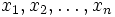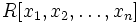# Multivariate polynomial ring

This is a variation of polynomial ring
View a complete list of variations of polynomial ring OR read a survey article on varying polynomial ring

## Definition

Let$R$ be a commutative unital ring. The$n$-variate polynomial ring over$R$ is defined as the ring of polynomials in$n$ symbols. If the$n$ symbols are$x_1, x_2, \ldots, x_n$, then the polynomial ring is$x_1, x_2, \ldots, x_n$.

The$n$-variate polynomial ring can be obtained by applying the polynomial ring operator$n$ times in succession.

When we simply say multivariate polynomial ring, we usually mean multivariate polynomial ring over a field.

We can also consider the polynomial ring in infinitely many variables over$R$.

## Extra structure

The multivariate polynomial ring over a ring$R$ is, first and foremost, a commutative unital ring. However, it has a number of additional structures, as described below.

### As an algebra over the original ring

The polynomial ring$R[x_1,x_2,\ldots,x_n]$ naturally gets the structure of a$R$-algebra. In fact it is free in the category of$R$-algebras, on$n$ generators.

A similar statement holds for polynomial rings in infinitely many variables.

The polynomial ring$R[x_1,x_2,\ldots,x_n]$ naturally gets the structure of a connected graded$R$-algebra (and hence a graded ring). The$d^{th}$ graded component is the free$R$-module spanned by all monomials of total degree$d$.
The polynomial ring$R[x_1,x_2,\ldots,x_n]$ naturally gets the structure of a connected filtered$R$-algebra (and hence a filtered ring). The$d^{th}$ filtered component is the subgroup comprising polynomials of degree at most$d$.